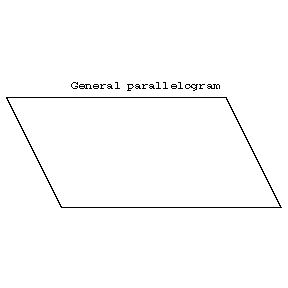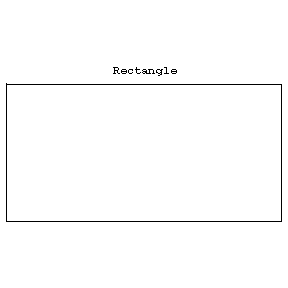# Parallelogram

A quadrangle with pairwise parallel sides (see Fig.a–Fig.d). Parallelograms can also be characterized as convex quadrangles satisfying any of the following conditions: 1) the first and the second pair of opposite sides consist of equal segments; 2) one pair of opposite sides consists of equal and parallel segments; 3) at opposite vertices, the first and the second pair of opposite angles is equal; or 4) the point of intersection of the diagonals bisects each of them. Various types of parallelograms are: a rectangle (b) — a parallelogram one angle, and hence all angles, of which is a right angle; a rhombus (c) — a parallelogram all sides of which are equal; a square (d) — an equal-sided rectangle.Figure: p071430aFigure: p071430bFigure: p071430cFigure: p071430d